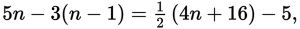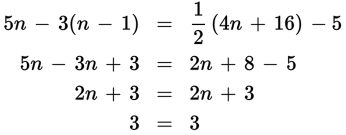# SAT Math Multiple Choice Question 430: Answer and Explanation

### Test Information

Question: 430

10. Ifwhat is the value of n?

• A. n = 1
• B. n = 3
• C. There is no value of n for which the equation is true.
• D. There are infinitely many values of n for which the equation is true.

Explanation:

D

Difficulty: Medium

Category: Heart of Algebra / Linear Equations

Strategic Advice: Use the distributive property to simplify each of the terms that contain parentheses. You don't need to clear the fractions on the right-hand side, because you can easily take half of 4n and half of 16. Once you arrive at the simplified equation, make sure you understand what the result is telling you.